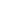GraduatesApplied mathematicsComputational mathematicsOperations Research and Control Theo...Probability and Mathematical Statist...Pure MathematicsStatistics

 UndergraduatesInformation & Computational SciencesMathematics & Applied Mathematics

 Research HighlightsAnalysis & GeometryComputational MathematicsOrdinary Differential Equations & Dy...Combinatorics & OptimizationStatistics